Share

# Construct δDef Such that De = 5 Cm, Df = 3 Cm and M∠Edf = 90°. - CBSE Class 7 - Mathematics

ConceptConstructing a Triangle When the Lengths of Two Sides and the Measure of the Angle Between Them Are Known. (Sas Criterion)

#### Question

Construct ΔDEF such that DE = 5 cm, DF = 3 cm and m∠EDF = 90°.

#### Solution

The rough sketch of the required ΔDEF is as follows.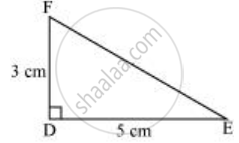The steps of construction are as follows.

1) Draw a line segment DE of length 5 cm.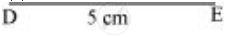2) At point D, draw a ray DX making an angle of 90° with DE.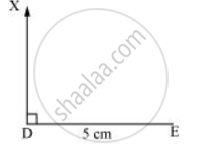3) Taking D as centre, draw an arc of 3 cm radius. It will intersect DX at point F.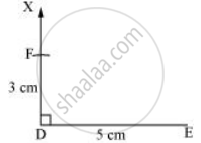4) Join F to E. ΔDEF is the required triangle.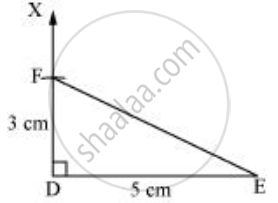Is there an error in this question or solution?

#### APPEARS IN

NCERT Solution for Mathematics for Class 7 (2018 to Current)
Chapter 10: Practical Geometry
Ex. 10.30 | Q: 1 | Page no. 200
Solution Construct δDef Such that De = 5 Cm, Df = 3 Cm and M∠Edf = 90°. Concept: Constructing a Triangle When the Lengths of Two Sides and the Measure of the Angle Between Them Are Known. (Sas Criterion).
S